### 13.1.2.2 A differential drive

Most indoor mobile robots do not move like a car. For example, consider the mobile robotics platform shown in Figure 13.2a. This is an example of the most popular way to drive indoor mobile robots. There are two main wheels, each of which is attached to its own motor. A third wheel (not visible in Figure 13.2a) is placed in the rear to passively roll along while preventing the robot from falling over.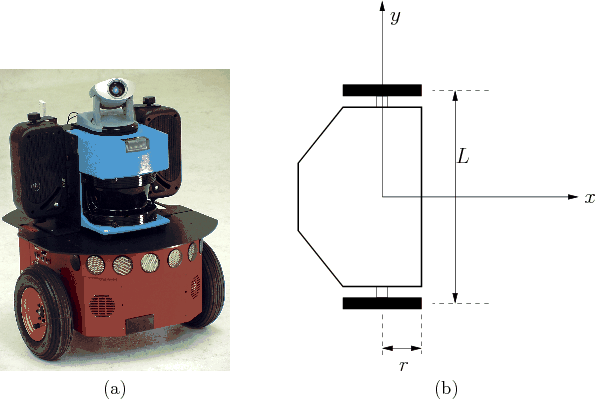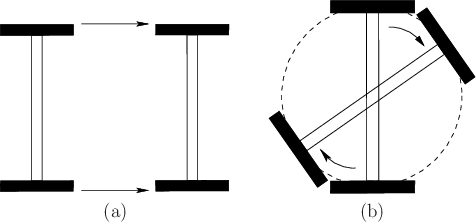To construct a simple model of the constraints that arise from the differential drive, only the distance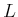between the two wheels, and the wheel radius,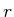, are necessary. See Figure 13.2b. The action vector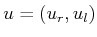directly specifies the two angular wheel velocities (e.g., in radians per second). Consider how the robot moves as different actions are applied. See Figure 13.3. If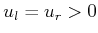, then the robot moves forward in the direction that the wheels are pointing. The speed is proportional to. In general, if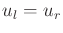, then the distance traveled over a duration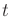of time is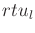(because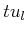is the total angular displacement of the wheels). If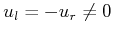, then the robot rotates clockwise because the wheels are turning in opposite directions. This motivates the placement of the body-frame origin at the center of the axle between the wheels. By this assignment, no translation occurs if the wheels rotate at the same rate but in opposite directions.

Based on these observations, the configuration transition equation is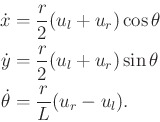(13.16)

The translational part contains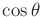and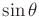parts, just like the simple car because the differential drive moves in the direction that its drive wheels are pointing. The translation speed depends on the average of the angular wheel velocities. To see this, consider the case in which one wheel is fixed and the other rotates. This initially causes the robot to translate at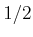of the speed in comparison to both wheels rotating. The rotational speed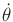is proportional to the change in angular wheel speeds. The robot's rotation rate grows linearly with the wheel radius but reduces linearly with respect to the distance between the wheels.

It is sometimes preferable to transform the action space. Let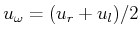and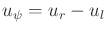. In this case,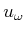can be interpreted as an action variable that means translate,'' and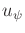means rotate.'' Using these actions, the configuration transition equation becomes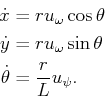(13.17)

In this form, the configuration transition equation resembles (13.15) for the simple car (try setting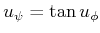and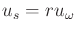). A differential drive can easily simulate the motions of the simple car. For the differential drive, the rotation rate can be set independently of the translational velocity. The simple car, however, has the speed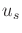appearing in theexpression. Therefore, the rotation rate depends on the translational velocity.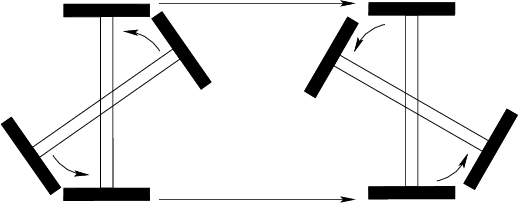Recall the question asked about shortest paths for the Reeds-Shepp and Dubins cars. The same question for the differential drive turns out to be uninteresting because the differential drive can cause the center of its axle to follow any continuous path in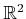. As depicted in Figure 13.4, it can move between any two configurations by: 1) first rotating itself to point the wheels to the goal position, which causes no translation; 2) translating itself to the goal position; and 3) rotating itself to the desired orientation, which again causes no translation. The total distance traveled by the center of the axle is always the Euclidean distance inbetween the two desired positions.

This may seem like a strange effect due to the placement of the coordinate origin. Rotations seem to have no cost. This can be fixed by optimizing the total amount of wheel rotation or time required, if the speed is held fixed . Suppose that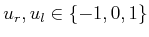. Determining the minimum time required to travel between two configurations is quite interesting and is covered in Section 15.3. This properly takes into account the cost of rotating the robot, even if it does not cause a translation.

Steven M LaValle 2020-08-14